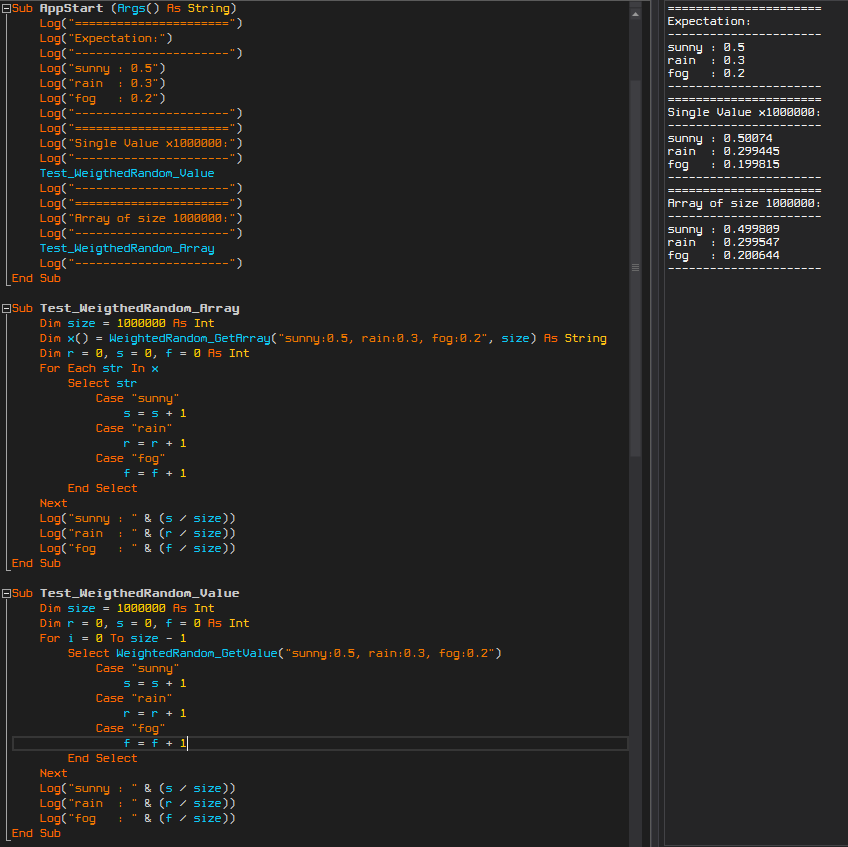# Android Code SnippetWeighted Random

Hi again!Say you are a game developer and you want to get a random weather condition where the probability of "sunny" is 50%, the probability of "rain" is 30% and the probability of "fog" is 20%.

WeightedRandom_GetValue("sunny:0.5, rain:0.3, fog:0.2")

Code:
B4X:
``````'Obtains an array of random values, based off the given inputs
Sub WeightedRandom_GetArray(weightMap As String, outputSize As Int) As String()
weightMap = weightMap.Replace(" ", "")
Dim output(outputSize) As String
Dim items() = Regex.Split(",", weightMap) As String
Dim lenItems = items.Length As Int
Dim keys(lenItems) As String
Dim vals(lenItems) As Int
'---------------------------------------------------
Dim rndUpperBound = 1 As Int
Do While lenItems / rndUpperBound > 1
rndUpperBound = rndUpperBound * 10
Loop
'---------------------------------------------------
Dim i = 0 As Int
Do While i < lenItems
Dim kv() = Regex.Split(":", items(i)) As String
keys(i) = kv(0)
vals(i) = kv(1) * rndUpperBound
i = i + 1
Loop
'---------------------------------------------------
Dim j = 0 As Int
Do While j < outputSize
i = 0
Dim rndValue = Rnd(0, rndUpperBound) As Int
Do While i < lenItems
If rndValue < vals(i) Then
output(j) = keys(i)
Exit
End If
rndValue = rndValue - vals(i)
i = i + 1
Loop
j = j + 1
Loop
'---------------------------------------------------
Return output
End Sub

'Obtains a random value, based off the given inputs
Sub WeightedRandom_GetValue(weightMap As String) As String
weightMap = weightMap.Replace(" ", "")
Dim items() = Regex.Split(",", weightMap) As String
Dim lenItems = items.Length As Int
'---------------------------------------------------
Dim rndUpperBound = 1 As Int
Do While lenItems / rndUpperBound > 1
rndUpperBound = rndUpperBound * 10
Loop
'---------------------------------------------------
Dim rndValue = Rnd(0, rndUpperBound) As Int
For Each item As String In items
Dim kv() = Regex.Split(":", item) As String
kv(1) = kv(1) * rndUpperBound
If rndValue < kv(1) Then Return kv(0)
rndValue = rndValue - kv(1)
Next
'---------------------------------------------------
Return Null
End Sub``````

Test Results (see attached):Notes:
- There is no error checking (reason: performance), it's up to you to implement it.
- You can easily change the input from a string to a map

#### Attachments

• Test WeightedRandom.zip
1.3 KB · Views: 220
Last edited:

#### Rantor777

##### Member
Very useful code...

Android Code Snippet Random Letter Generation
Replies
5
Views
4K
Replies
2
Views
672
B4A Library libGDX - Game Engine
Replies
517
Views
115K
Replies
82
Views
43K
Android Tutorial Converting VB6 to B4A
Replies
67
Views
107K